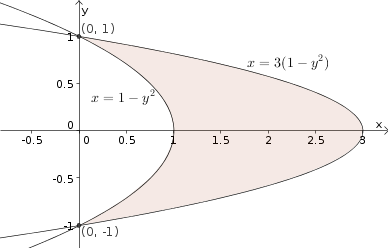# Math Insight

### Image: Example region bounded by parabolas for change of variablesTo evaluate an integral over the region bounded by the parabolas $x=1-y^2$ and $x=3(1-y^2)$, one can use the change of variables $(x,y) = \cvarf(\cvarfv,\cvarsv) = (\cvarsv(1-\cvarfv^2), \cvarfv)$.

Source image file: double_integral_change_variable_example_region_parabolas.ggb
Source image type: Geogebra